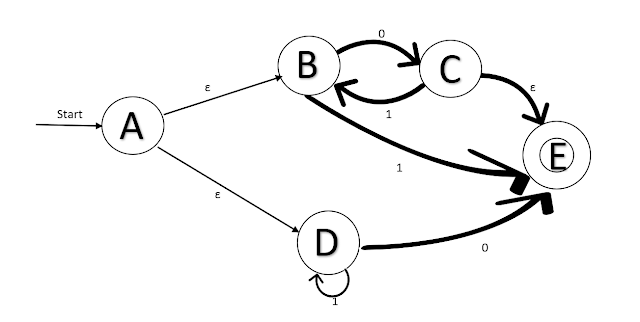# Eliminating Epsilon transition (ε-Transitions) | Conversion of Epsilon-NFA to DFA | Conversion of Epsilon-NFA to NFA | Theory Of Computation (TOC)### Theory Of Computation (TOC) | Eliminating Epsilon Transition(ε-Transitions):

Given any Epsilon NFA (ε-NFA) , we can ﬁnd a DFA D that accepts the same language as E. The construction is close to the subset construction, as the states of D are subsets of the states of E. The only difference is that dealing with ε-transitions, which can be done by using ε-closure.

Epsilon NFA (ε-NFA) - DFA:

Let E = (QE,∑, 𝛿E,qo, FE) is ε-NFA. The DFA equivalent to given ε-NFA is constructed as:

D = (QD,, 𝛿D,qD, FD)

Where,

QD= set of subsets of QE i.e QD ⊆ 2ᣔQE
qD=ε-closure(qo)
FD = set of states that contains at least one accepting state of E.
i.e. FD= {S|S is in qD and S∩FE∉φ}.
𝛿D (S, a) is computed, for all a in and sets S in QD by:

• Let 𝛿cap(q, x) = {p1, p2,......, pk} i.e. pi ’s are the states that can be reached from q following path labeled x which can end with many ε & can have many ε.
• Compute

• Then,

For Example:

### Conversion of Epsilon-NFA to DFA

Given an ε-NFA as:

### ε-NFA —NFA:

Let E = (QE,∑, 𝛿E,qo, FE) is ε-NFA. The NFA equivalent to given ε-NFA is constructed as:
N = (QN,, 𝛿N,qN, FN)

• Start state of NFA =Start state of ε-NFA i.e. qN= qo
• QN= set of subsets of QE i.e QN ⊆ QE
• For any state q  ∈ QN and a ∈ Σ:
𝛿N(q, a) = ε-closure (𝛿E(ε- closure(q), a))
• FN= { q ∈ QN: ε- closure(q) ∩FE≠φ}

#### For Example:

Given an ε-NFA as:

#### Transition Diagram:

1.